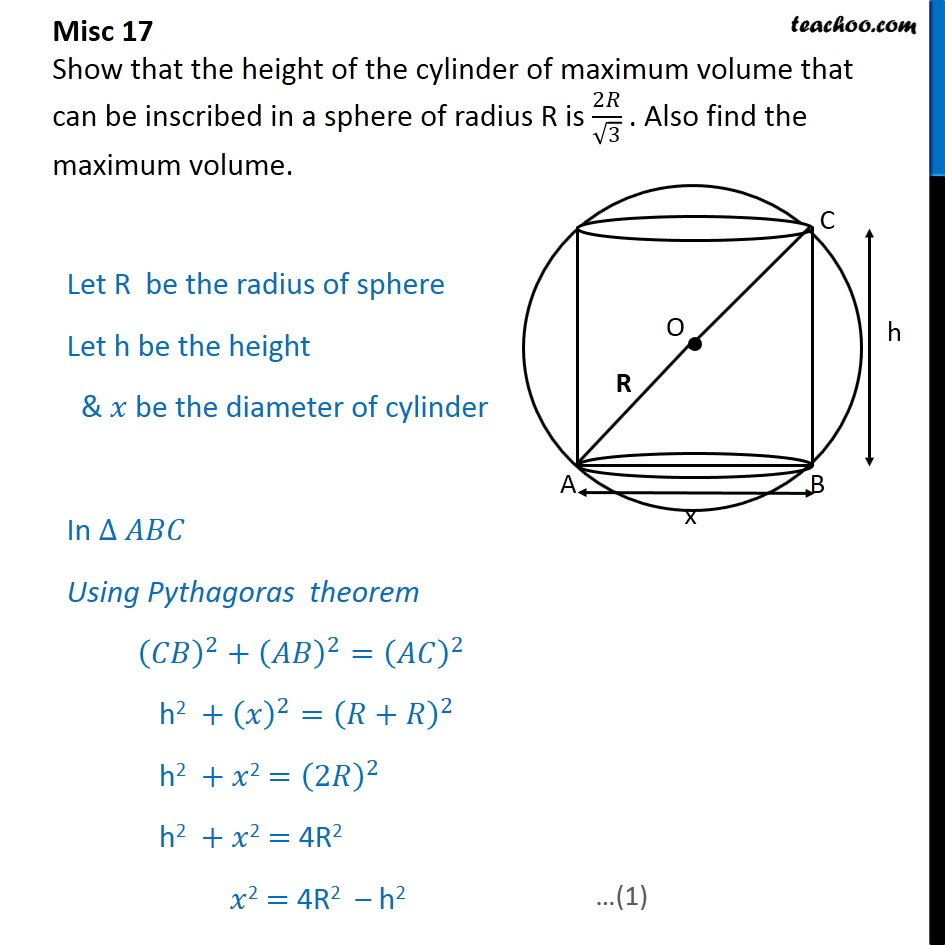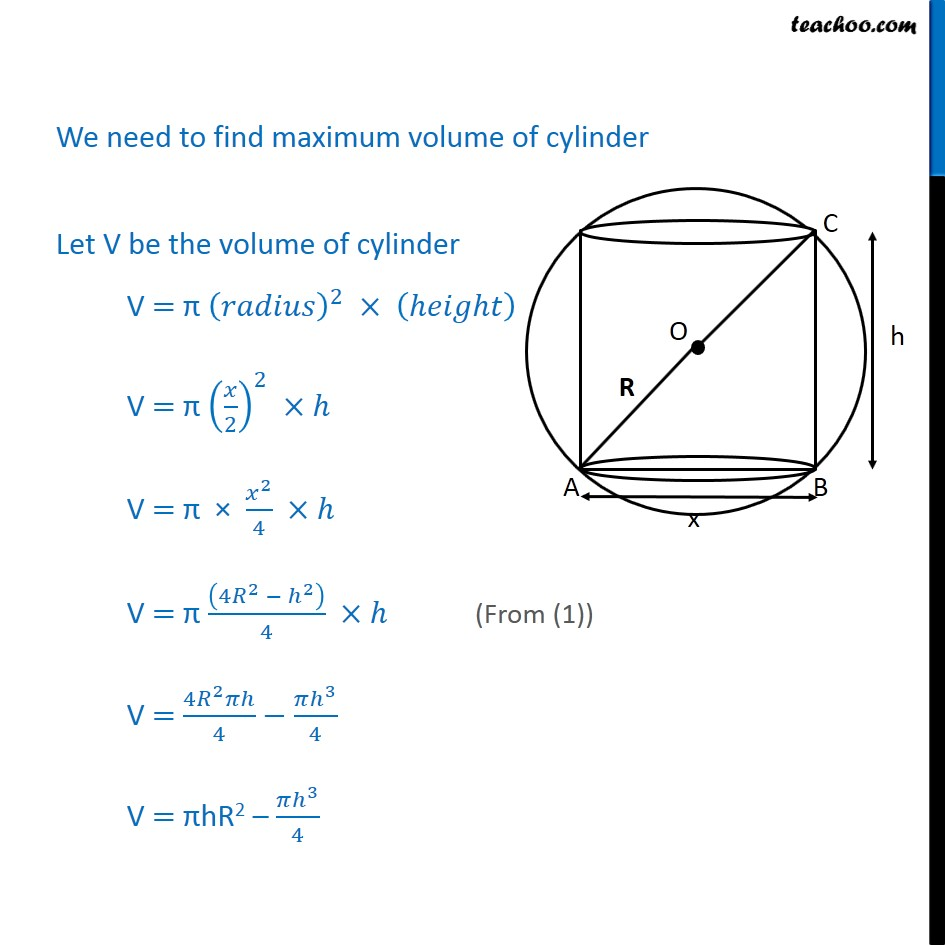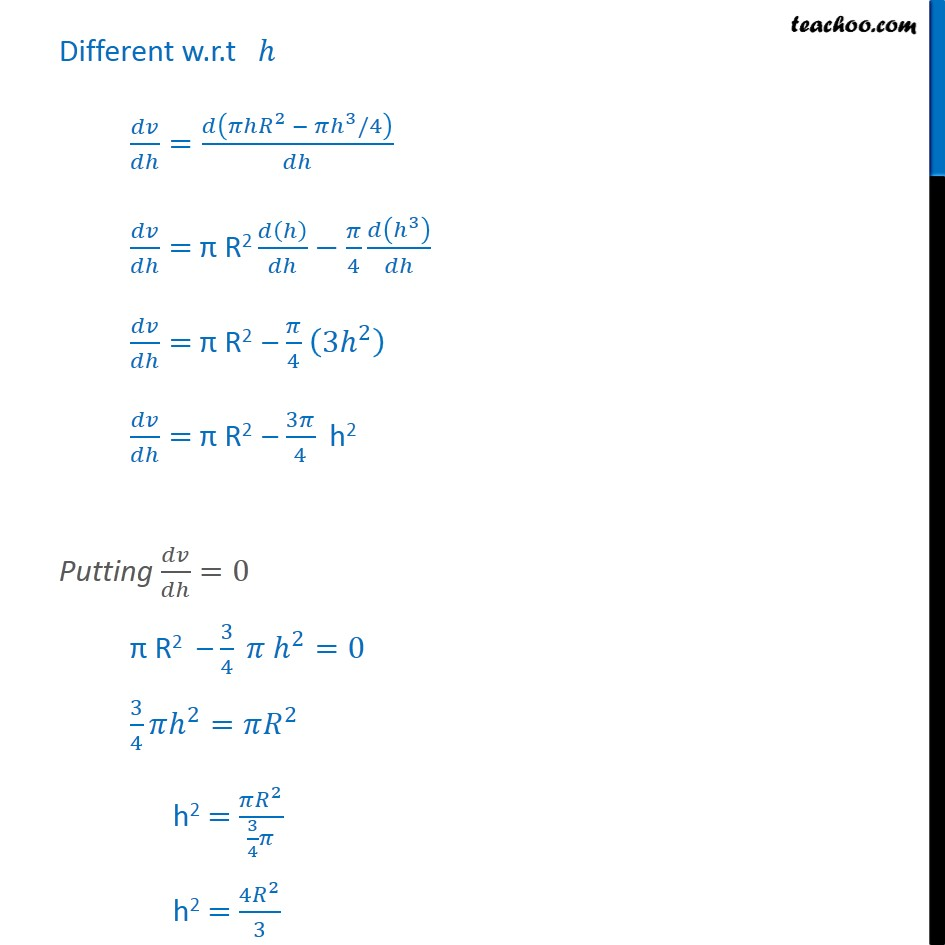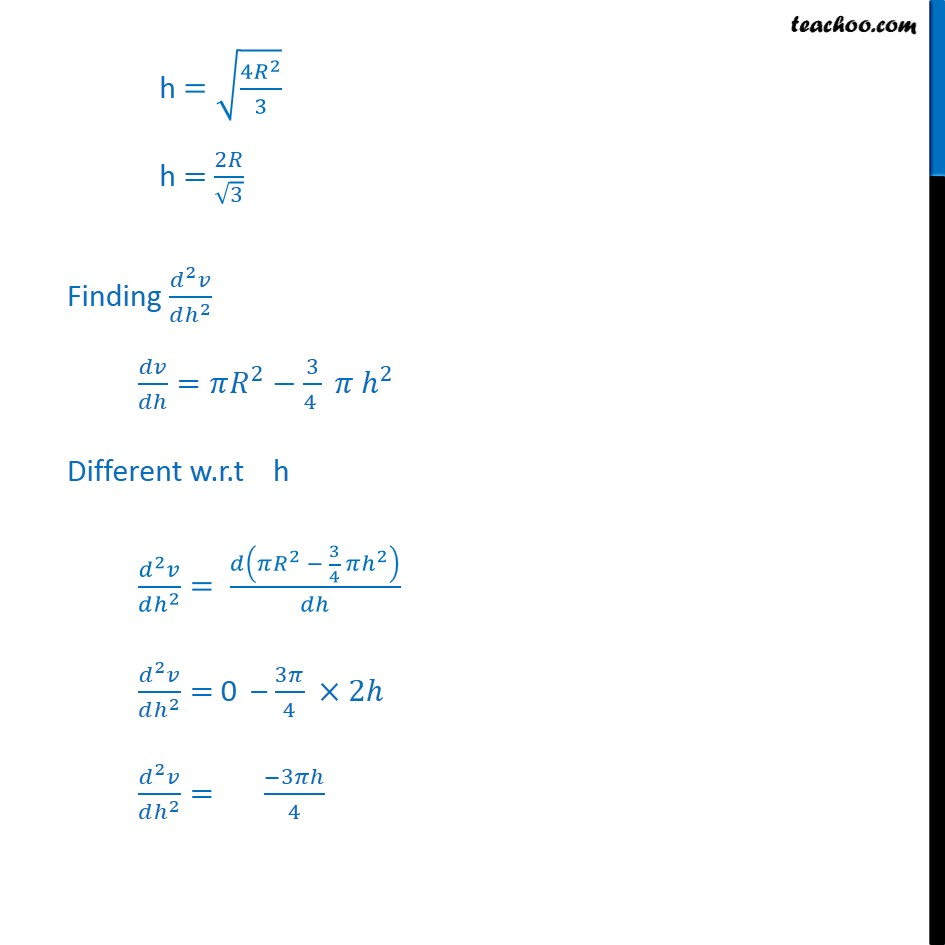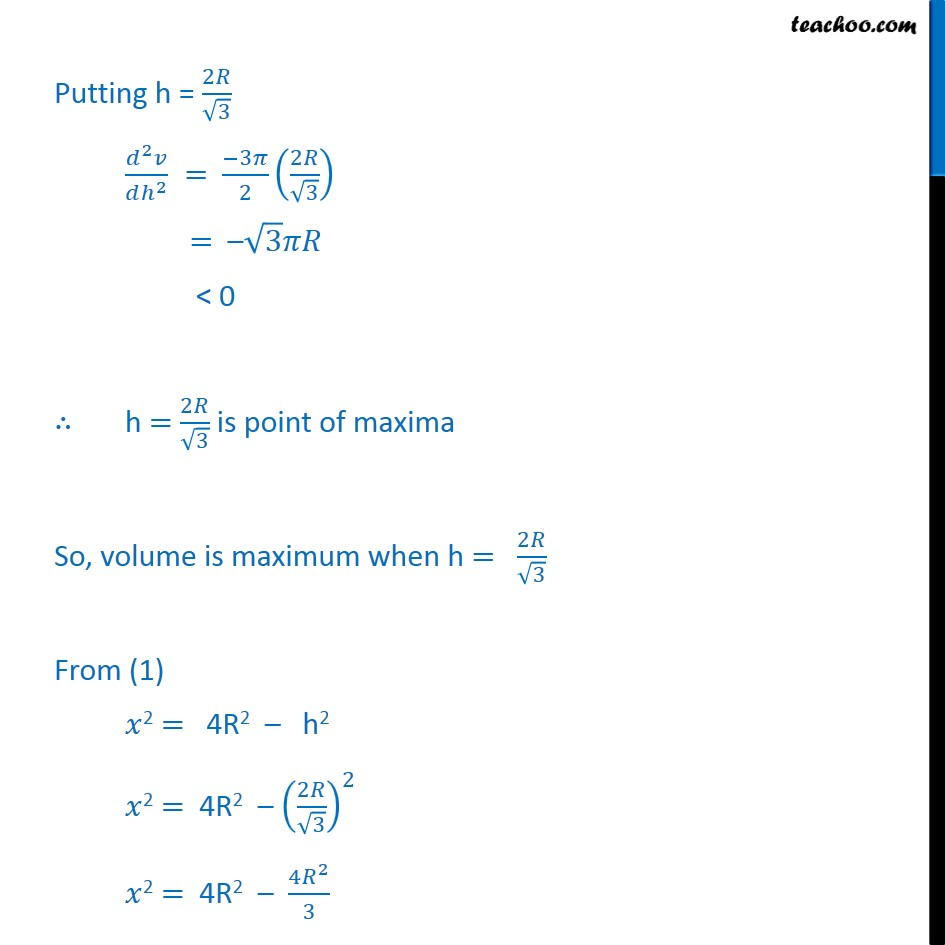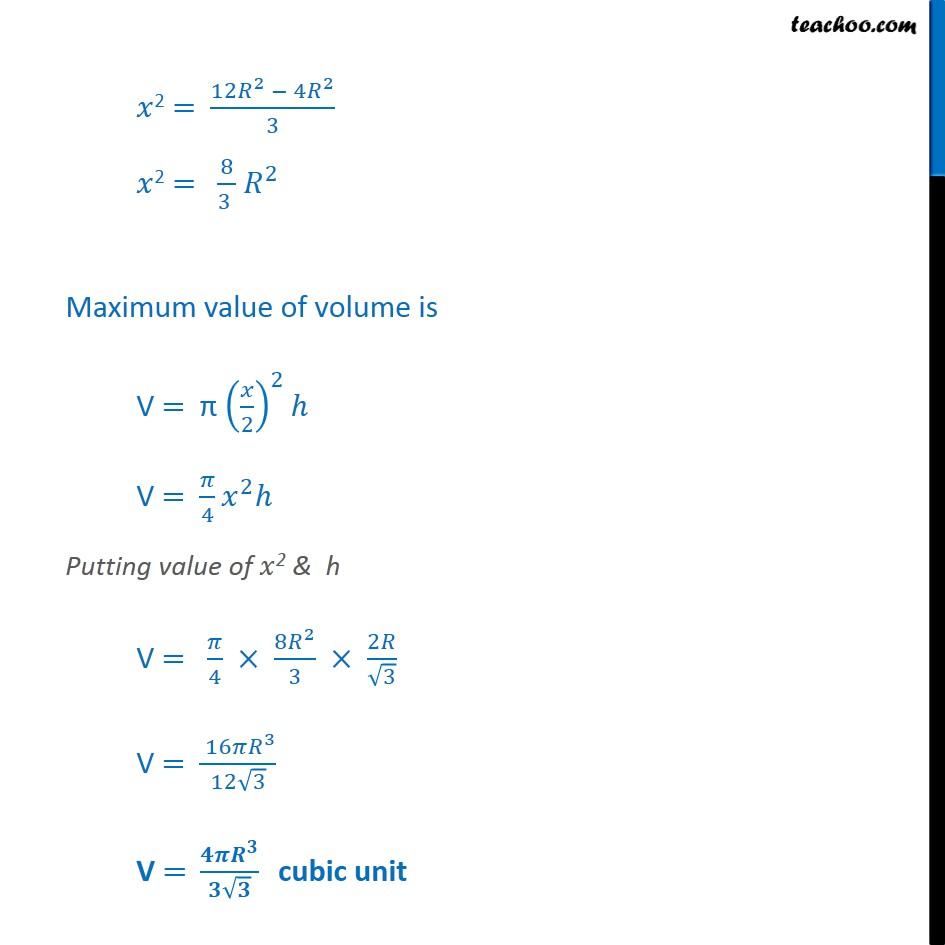1. Class 12
2. Important Question for exams Class 12
3. Chapter 6 Class 12 Application of Derivatives

Transcript

Misc 17 Show that the height of the cylinder of maximum volume that can be inscribed in a sphere of radius R is 2 3 . Also find the maximum volume. Let R be the radius of sphere Let h be the height & be the diameter of cylinder In Using Pythagoras theorem 2 + 2 = 2 h2 + 2 = + 2 h2 + 2 = 2 2 h2 + 2 = 4R2 2 = 4R2 h2 We need to find maximum volume of cylinder Let V be the volume of cylinder V = 2 V = 2 2 V = 2 4 V = 4 2 2 4 V = 4 2 4 3 4 V = hR2 3 4 Different w.r.t = 2 3 /4 = R2 4 3 = R2 4 3 2 = R2 3 4 h2 Putting =0 R2 3 4 2 =0 3 4 2 = 2 h2 = 2 3 4 h2 = 4 2 3 h = 4 2 3 h = 2 3 Finding 2 2 = 2 3 4 2 Different w.r.t h 2 2 = 2 3 4 2 2 2 = 0 3 4 2 2 2 = 3 4 Putting h = 2 3 2 2 = 3 2 2 3 = 3 < 0 h = 2 3 is point of maxima So, volume is maximum when h = 2 3 From (1) 2 = 4R2 h2 2 = 4R2 2 3 2 2 = 4R2 4 2 3 2 = 12 2 4 2 3 2 = 8 3 2 Maximum value of volume is V = 2 2 V = 4 2 Putting value of 2 & h V = 4 8 2 3 2 3 V = 16 3 12 3 V = cubic unit

Chapter 6 Class 12 Application of Derivatives

Class 12
Important Question for exams Class 12

About the AuthorDavneet Singh
Davneet Singh is a graduate from Indian Institute of Technology, Kanpur. He has been teaching from the past 9 years. He provides courses for Maths and Science at Teachoo.